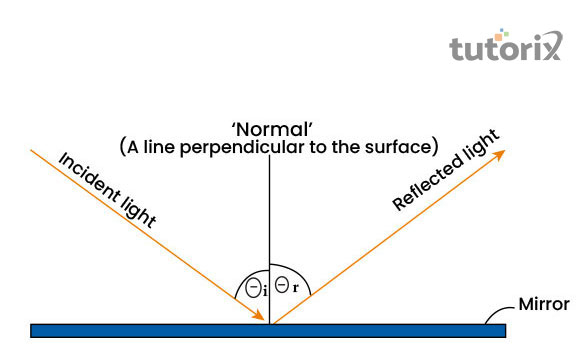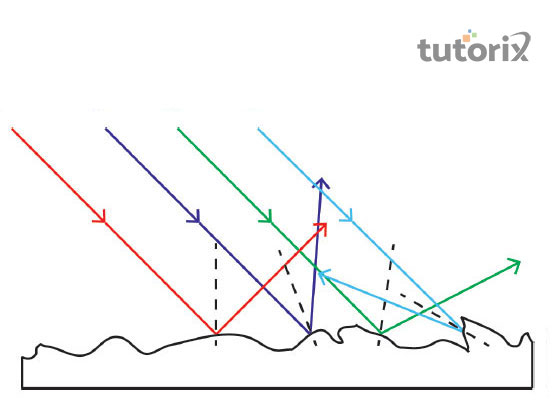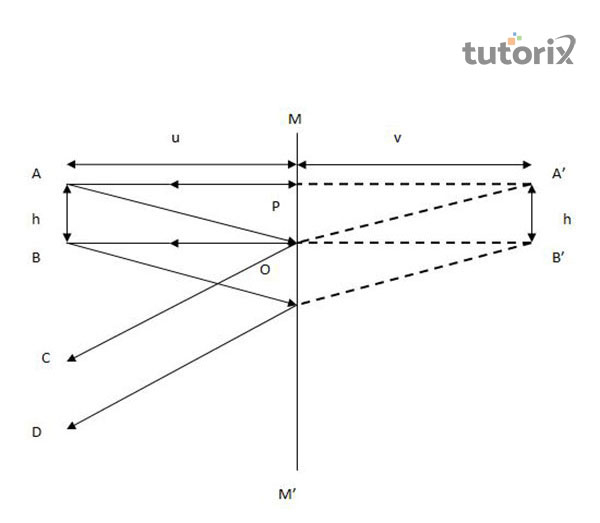# Reflection On A Plane Mirror

## Introduction

The present tutorial will explain the reflection and the laws of reflection that helps to create a mirror image after the ray of light bounces on the surface of a flat mirror. The tutorial will further explain the classification of lights along with the laws including Huygens Principle and Law of Refraction. Along with that, the tutorial will include how the angle of reflection changes for a rotational in comparison to a fixed mirror.

## What Is The Reflection of Light?

Reflection can be defined as the result of a change in direction of the angle of light or the wave front that takes place at two different interface media. As a consequence of such a phenomenon, the wave front returns to the source media from where the wave front has originated (Splung, 2022).

After being stroked on the flat surface of a mirror, the ray of light is reflected back.

## Classification of Reflection

Two types of classification of reflection are found. The types of these two classifications of reflection are as follows:

### Specular reflectionFigure 1: Specular reflection

This specific kind of reflection shares similarities to the way a mirror reflects rays of light. After being originated from a source object, the rays of light hits the upper surface of a plane object like a mirror that creates a reflection of the rays at a definitive angle. Here the angle of the reflected ray is similar to the incident ray.

### Diffuse reflectionFigure 2: Diffuse reflection

All the rays of light that hit the surface of the reflector are not always reflected in a direction that is definite. While reflecting light if the reflectors' surface is identified as rough, then the outgoing rays are reflected at different angles as the incident rays hit different regions of the surface at different angles.

## Laws of Reflection

The first law of reflection states that at the point of the incident, rays of incident reflected and normal lie on a similar plane. This particular law leads to the development of the second law of reflection. The second law of reflection states that the angle of incidence will be always equal to the angle of reflection (Zhang et al. 2021). This second law of reflection can be displayed as a formula that is θi = θr. The angle of the incident ray and the reflected ray form a 90° angle with the surface.

## Nature of Image Formation by a Plane MirrorFigure 3: Image Formation by a Plane Mirror

The first aspect of the reflected image of a mirror is that the image will be erect and virtual. As per the discussion of the second law of reflection, the image that will be formed at the other end of the mirror will have a similar distance from the mirror and size to the source image (Shaalaa, 2022). The reflection will be formed behind the mirror and this formed image will be laterally inverted. The phrase laterally inverted means that if one person, standing before the mirror, raises his left hand, in the mirror image it will appear that the person has raised the right hand.

## Reflection of the Flat Mirror and Rotational Mirror

In the mirror that is fixed, the reflected angle and the angle of striking of ray on the surface are the same that establishing the formula θi = θr. In such cases, without changing the other aspects, if the mirror surface is roasted through an angle φ, the angle of the incident will be increased to θ +φ (Splung, 2022). Based on this, the angle of reflection also increased from φ to θ+φ. In such cases, the difference between the final and initial angle will be 2φ.

## Huygen's Principle and Law of Refraction

Huygen's Principle states that the surface named wave front can be drawn between any in phase points on two or more waves. The wave fronts will be always perpendicular in direction to the waves (Wang, 2018). Moreover, this principle states that any number of wavefronts wil be considered as the sources of wavelets. In such cases. The wavelets will be spherical that is expanded outwards at the same speed.

## Conclusion

The tutorial has cast light on providing effective and understandable information regarding the reflection of light on the flat and plain mirror. The laws of reflection have been included in the explanation part along with the two major types of reflection witnessed naturally such as specular reflection and diffused reflection. Regular reflection is largely known as specular reflection and shares similarities with mirror-like reflection whereas irregular or effusive reflection refers to a non-mirror type reflection of the light ray.

## FAQs

Q1. What is the angle of incidence?

The angle of incidence can b defined by the particular angle that is made by a ray of light or wave after hitting on the surface and reflection makes a perpendicular line to that surface.

Q2. What is the relation between object distance and image distance in mirrors?

In the case of a flat or plane mirror, the distance of the image that is reflected shares a similar range of distance to the object. For instance, if a person stands at a distance of 3 meters from the plan mirror the distance of the mirror image will be formed at a similar distance.

Q3. Why does a shiny surface need the creation of a reflection?

In the case of a rough surface, there is a possibility of having scattered images as the reflected ray will go in multiple directions in a non-parallel manner whereas, a polished surface will reflect light in a proper manner that will generate a clear image.

Q4. What factors affect the amount of reflected light?

Two factors such as the degree of the surface where the light will bounce and the texture of that surface affects the amount of reflected light.

## References

### Journals

Wang, P. (2018). Geometrical ray tracing in uniaxial crystals: direct formulas and the equivalence between the electromagnetic wave method and Huygens' principle. JOSA A, 35(7), 1114-1123. Retrieved from: https://opg.optica.org

Zhang, J., Donaldson, W. R., & Agrawal, G. P. (2021). Temporal reflection and refraction of optical pulses inside a dispersive medium: an analytic approach. JOSA B, 38(3), 997-1003. Retrieved from: https://www.lle.rochester.edu

## Websites

Photokonnexion.com, (2022). Definition: Specular Reflection. Retrieved from: https://www.photokonnexion.com [Retrieved on 10th June 2022]

Shaalaa.com, (2022). Describe the nature of images formed by plane mirrors. Retrieved from: https://www.shaalaa.com [Retrieved on 10th June 2022]

Splung.com, (2022). Geometric Optics. Retrieved from: http://www.splung.com [Retrieved on 10th June 2022]